# Parent-Child HierarchiesDAX does not directly support parent-child hierarchies. To obtain a browsable hierarchy in the data model, you have to naturalize a parent-child hierarchy. DAX provides specific functions to naturalize a parent-child hierarchy using calculated columns. The complete pattern also includes measures that improve the visualization of ragged hierarchies in Power Pivot.

## Basic Pattern Example

Suppose you have an Agents table containing sales figures for each agent. The Parent column specifies the direct report of each agent, as you see in Figure 1.

You might represent relationships between nodes using a tree structure, where all nodes without a parent are roots of a hierarchy tree, as shown in Figure 2.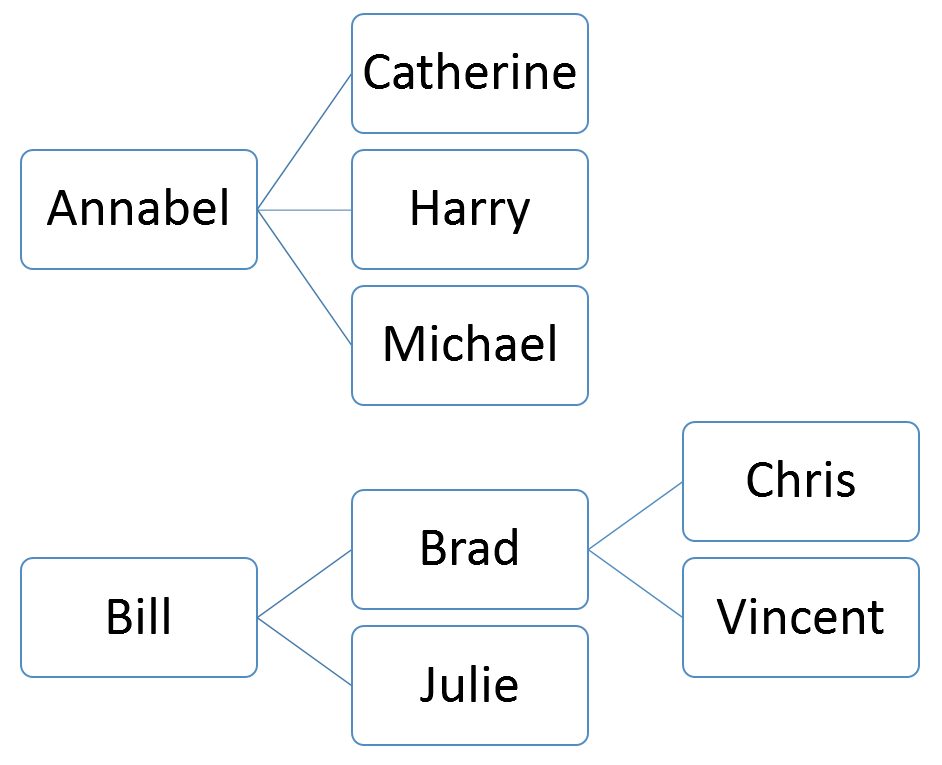Figure 2 The hierarchy has two branches—one with two levels and one with three levels.

Your goal is to create one calculated column for each level of the hierarchy. To create the right number of calculated columns, you must know in advance the maximum depth of the hierarchy. Otherwise, you have to estimate it, because this number cannot change dynamically. Power Pivot and Analysis Services hierarchies have an intrinsic limit of 64 levels.

Figure 3 shows the resulting table with the naturalized hierarchy.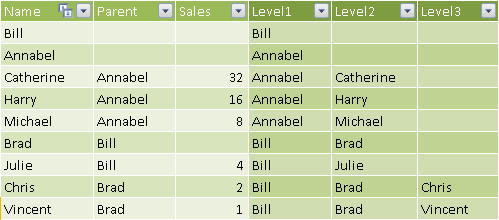Figure 3 The naturalized hierarchy has one column for each level of the hierarchy.

You can create these columns in DAX by leveraging a hidden calculated column that provides a string with the complete path to reach the node in the current row of the table. The Path column in Figure 4 provides this content using the special PATH function.

```[Path] = PATH ( Nodes[Name], Nodes[Parent] )
```

Each column that defines a level in the hierarchy uses the PATHITEM function to retrieve the proper value for that level, as shown in Figure 3.

```[Level1] = PATHITEM ( Nodes[Path], 1 )

[Level2] = PATHITEM ( Nodes[Path], 2 )

[Level3] = PATHITEM ( Nodes[Path], 3 )
```

You define the hierarchy in the diagram view of the data model shown in Figure 5.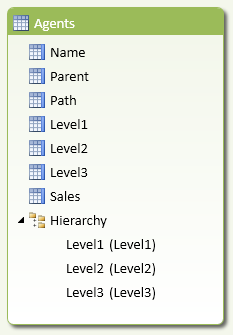Figure 5 The Hierarchy heading includes the calculated columns created to naturalize the hierarchy.

You can navigate the resulting hierarchy in Excel as shown in Figure 6.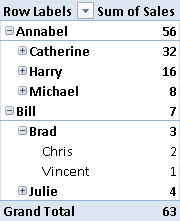Figure 6 The Sum of Sales measure displays the total sales of all agents below the selected node in the hierarchy.

You can browse a pivot table and navigate this hierarchy down to the third level. If an intermediate node of the hierarchy has no children, you can still drill-down to an empty label, although this would result in a row of the pivot table with no description. You can avoid this behavior in Analysis Services Tabular by using the HideMemberIf property, and in Power Pivot by using the technique described in the complete pattern.

## Use Cases

You can use the Parent-Child Hierarchies pattern any time you have a corresponding structure in your source data. This is a list of common use cases.

### Profit and Loss Account Hierarchy

Most profit and loss statements have a native parent-child hierarchy for representing the list of accounts. When this is not the native representation in the data source, a parent-child hierarchy can be useful to show an alternative custom grouping of original accounts, such as in balance sheet reclassification.

### Bill of Materials

A list of components of a product is usually a native parent-child hierarchy, because each component has other subcomponents, with different levels of depth in different branches of the hierarchy. Calculations related to measures in a bill of materials are described in another dedicated pattern, Bills of Materials.

### Organizational Structure

Company organizational structures are often represented as parent-child hierarchies. One of the limitations of parent-child hierarchies is that each node must have a single parent. Complex organizations that do not respect this constraint require more complex graphs, and mapping them to a parent-child hierarchy requires a normalization to a regular organization tree.

## Complete Pattern

Suppose you have a Nodes table containing one row per node, with a ParentKey column that defines the parent of every node. The Transactions table has a many-to-one relationship with the Nodes table. You can see the two tables in Figure 7.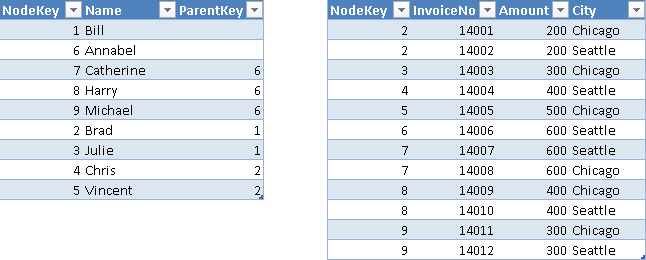Figure 7 The Nodes table defines a parent-child hierarchy through the NodeKey and ParentKey columns.

You create a hidden HierarchyPath column containing the result of the PATH function, which provides a string with the complete path to reach the node in the current row of the table, as shown in Figure 8.

```[HierarchyPath] = PATH ( Nodes[NodeKey], Nodes[ParentKey] )
```

You naturalize the hierarchy by creating a hidden column for each level of the hierarchy. You have to define the maximum depth of the hierarchy in advance, planning enough levels for future growth. For each level, you populate the column with the node name of the hierarchy path at that level. You need to duplicate the name of the leaf node if the level is higher than the number of levels in the hierarchy path, which you obtain in the HierarchyDepth column using the PATHLENGTH function.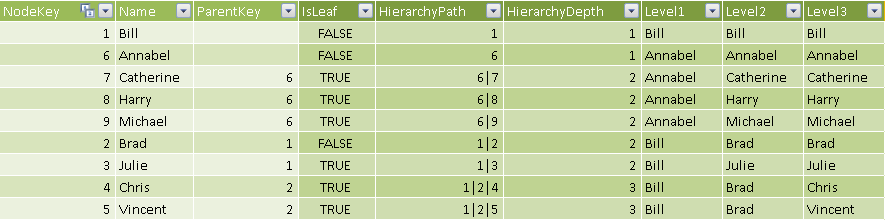Figure 9 The HierarchyDepth column defines the level of the node in the current row.

In Figure 9 you can see the resulting column for the levels of the hierarchy, populated using the LOOKUPVALUE and PATHITEM functions. The hierarchy path is a string, but the LOOKUPVALUE has to match an integer column, so you need to cast the hierarchy path value to INT using the third argument of PATHITEM. The following formulas are used for the calculated columns in Figure 9.

```[HierarchyDepth] = PATHLENGTH ( Nodes[HierarchyPath] )

[Level1] =
LOOKUPVALUE (
Nodes[Name],
Nodes[NodeKey],
PATHITEM ( Nodes[HierarchyPath], 1, INTEGER )
)

[Level2] =
IF (
Nodes[HierarchyDepth] >= 2,
LOOKUPVALUE (
Nodes[Name],
Nodes[NodeKey],
PATHITEM ( Nodes[HierarchyPath], 2, INTEGER )
),
Nodes[Level1]
)

[Level3] =
IF (
Nodes[HierarchyDepth] >= 3,
LOOKUPVALUE (
Nodes[Name],
Nodes[NodeKey],
PATHITEM ( Nodes[HierarchyPath], 3, INTEGER )
),
Nodes[Level2]
)
```

In order to hide nodes duplicated at lower levels while browsing hierarchy in a pivot table, you create an IsLeaf calculated column, which contains a flag for nodes that have no children in the parent-child hierarchy, as you see in Figure 10.

```[IsLeaf] =
CALCULATE (
COUNTROWS ( Nodes ),
ALL ( Nodes ),
Nodes[ParentKey] = EARLIER ( Nodes[NodeKey] )
) = 0
```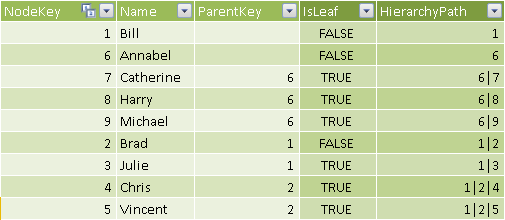Figure 10 The IsLeaf column marks as TRUE the nodes that have no children in the hierarchy.

The naturalized hierarchy duplicates leaf-level nodes that you do not want to display in a pivot table. In an Analysis Services Tabular model, you can hide these nodes by setting the HideMemberIf property with BIDS Helper. In Power Pivot, you have to build a DAX measure that returns a blank value for a “duplicated” node. You create two hidden measures to support such a calculation: BrowseDepth calculates the level of the hierarchy displayed in a pivot table, and MaxNodeDepth returns the maximum depth of the original parent-child hierarchy starting at the node considered. When BrowseDepth is higher than MaxNodeDepth, the node value should be hidden in the pivot table. You can see in Figure 11 a comparison of the BrowseDepth and MaxNodeDepth values returned for each node of the naturalized hierarchy.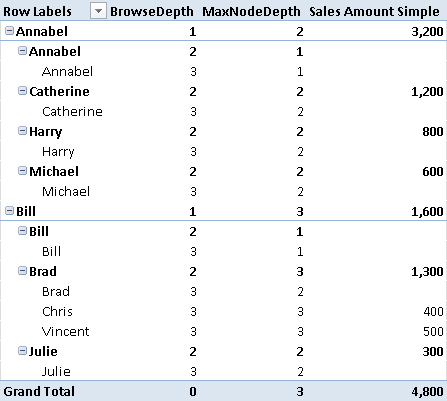Figure 11 Nodes having BrowseDepth higher than MaxNodeDepth might not have data to display.

The Sales Amount Simple measure in Figure 11 displays blank when BrowseDepth value is higher than MaxNodeDepth.

```[Sales Amount Simple] :=
IF (
[BrowseDepth] > [MaxNodeDepth],
BLANK (),
SUM ( Transactions[Amount] )
)
```

If you display such a measure in a pivot table with default settings (hiding empty rows), you will see a result like Figure 12.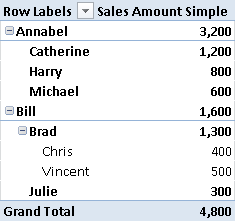Figure 12 The pivot table hides nodes for which the Sales Amount Simple measure returns a blank value.

The Sales Amount Simple measure does not display a separate value for intermediate nodes that have values associated both with children and with the node itself. For example, in Figure 12 you can see that the value related to Annabel is higher than the sum of her children, and the same happens for the value related to Brad. The reason is that both Annabel and Brad have directly related transactions. You can show the value for these nodes by implementing a more complex test, checking whether a leaf node has related transactions. The final Sales Amount measure considers all of these conditions, and its result is shown in Figure 13.

```[Sales Amount] :=
IF (
[BrowseDepth] > [MaxNodeDepth] + 1,
BLANK (),
IF (
[BrowseDepth] = [MaxNodeDepth] + 1,
IF (
AND (
VALUES ( Nodes[IsLeaf] ) = FALSE,
SUM ( Transactions[Amount] ) <> 0
),
SUM ( Transactions[Amount] ),
BLANK ()
),
SUM ( Transactions[Amount] )
)
)
```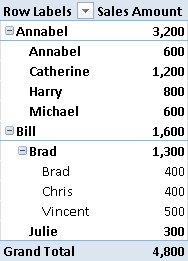Figure 13 The Sales Amount measure displays values associated with intermediate nodes, such as Annabel and Brad.Enclose code in comments with <PRE></PRE> to preserve indentation.